# Solving Linear Systems

Problem

You'd like to solve a linear system of equations using Excel.

Solution

You can take one of several approaches to solving linear systems in Excel. You could, for example, use VBA to program any of the standard numerical algorithms for solving systems of equations. While this approach would undoubtedly work, it's the most time-consuming since you'd have to program and test the algorithm. Since Excel provides built-in support for matrix operations, you could frame the problem in the form of a matrix problem and perform the necessary matrix inversion to find a solution. An easier way is to use Solver. In the discussion that follows, I'll show you the latter two approaches.

Discussion

If you can frame the system of equations in the form of a matrix equation, then you can use direct matrix inversion to find a solution. If you can't or don't want to do so, or if you're dealing with nonlinear equations, you can use one of Solver's several approaches.

Matrix inversion

In Recipe 7.10, I showed you how to use Excel's built-in matrix functions to multiply and invert matrices. You can use these same functions to solve a linear system of equations of the form: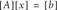The approach to solving this problem is a straightforward application of the built-in matrix functions, where you can find the unknown xs that satisfy this equation by solving the following matrix equation: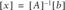For example, let's say you're given [A] and [b] matrices as shown in Figure 9-12.

Figure 9-12. Solving a matrix equation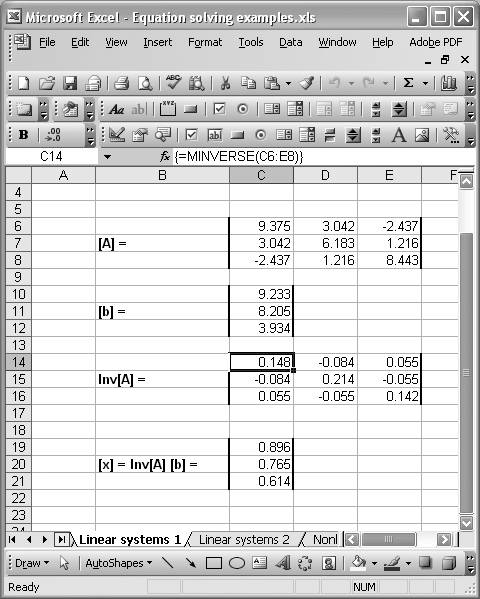The first step in the solution is to invert the [A] matrix. To do so, enter the matrix formula =MINVERSE(C6:E8). Remember, this is a matrix formula entered in a range of cells; you have to press Ctrl-Shift-Enter to enter the formula. The results of this matrix inversion are shown in Figure 9-12 in cells C14 through E16.

The next and final step is to multiply the inverse of the [A] matrix by the [b] matrix using the formula =MMULT(C14:E16,C10:C12). Here again, this is a matrix formula entered in a range of cells. The final results are shown in Figure 9-12 in cells C19 through C21.

Using Solver with constraints

Instead of using matrix functions, which can get a little unwieldy for large matrices, you can use Solver to solve a linear system (or a nonlinear one, as discussed in Recipe 9.5). In this example, I'll show you how to solve the same linear system discussed earlier by using Solver with constraints. Figure 9-13 shows how this problem is set up.

Figure 9-13. Solving a linear system with Solver by using constraintsThis problem essentially deals with three equations and three unknowns, the xs. The equations are of the form a1x1 + a2x2 + a3x3 = b. In this approach, I consider the system in this equation form rather than in the matrix form shown earlier. It's the same system, just with different notation and format.

The first thing I do in this approach is set up a table containing the given a-coefficients and b-coefficients along with initial guesses for the corresponding xs. Referring to Figure 9-13, I entered the a-coefficients in cells D9 through F11, the b-coefficients in cells I9 through I11, and the xs in cells H9 through H11.

Next, I set up a column of cells (J9 through J11) containing formulas of the form =D9*\$H\$9+E9*\$H\$10+F9*\$H\$11. These formulas compute b for each equation, given the a-coefficients and the initial guesses for the xs. Ideally, the results in this column should equal the given b-values shown in the adjacent column. Since initially the xs are only guesses, the b-results will differ. However, we can use Solver to find xs that will make these two columns of b-values the same, thus resulting in the solution to this system of equations.

Open Solver by selecting ToolsSolver...from the main menu bar. Instead of maximizing, minimizing, or setting a target value for some target cell, were going to leave the target cell blank, as shown in Figure 9-14.

Figure 9-14. Solver with constraints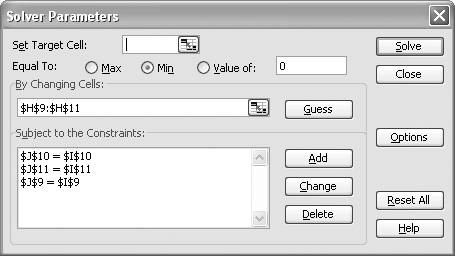We will set the By Changing Cells fields to the range of cells containing the x-values, cells H9 through H11 in this case. Now it's time to add some constraints.

Basically, we want to let Solver vary the xs subject to the constraints that the computed b-values in cells J9 through J11 are equal to the given b-values in cells I9 through I11. So we have to add a constraint for each b-value.

Figure 9-14 shows the constraints I set up in Solver. To add a constraint, press the Add button to open the Add Constraint window shown in Figure 9-15.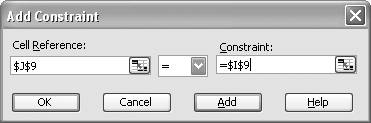In the Cell Reference field, select one of the cells containing a computed b-value. Then in the Constraint field, select the cell containing the corresponding given b-value. In the drop-down listbox in the center of the window, select the equals sign (=). Press the Add button to add the constraint. Pressing Add does not close the Add Constraint window, so you can go ahead and add the other two constraints as well. Press Cancel when you're done to close the window. Upon doing so, you should see the constraints as shown in Figure 9-14.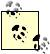You might be wondering why we didn't add a target cell. It turns out that Solver will add a dummy target cell if one is omitted. We can take advantage of that fact to simply add our constraints, since the actual value of the dummy cell is irrelevant to the solution.

After everything is set up, you can press Solve to actually solve the problem. Solver should quickly find a solution as in Figure 9-14, where the column of x-values (H9 through H11) represents the solution to the given system of equations. Notice that these x-values are equal to those found earlier using matrix inversion.

Using Solver to minimize residuals

An alternative approach to using constraints in Solver is to frame the problem in the form of minimizing residuals. We can easily do this by adding a column to the setup shown in Figure 9-14. The new setup is shown in Figure 9-16.

Figure 9-16. Solving a linear system with Solver by minimizing residuals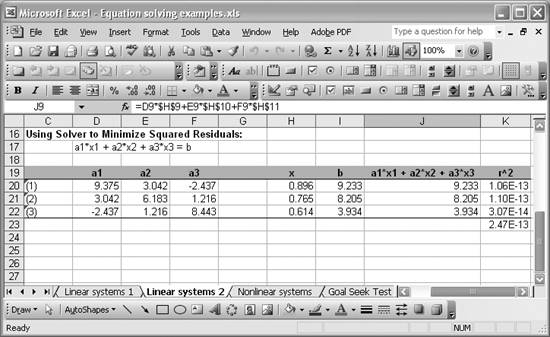Column K contains the square of residuals between the given b-values (column I) and the computed b-values (column J). The formulas in column K are of the form =(I20-J20)^2. Cell K23 contains the sum of these squared residuals, using the formula =SUM(K20:K22).

This is now essentially a least-squares problem, where we want to minimize the sum of squared residuals. We can readily use Solver to minimize this sum by changing the x-values contained in column H.

This is a straightforward application of Solver using techniques already discussed in this chapter. Figure 9-17 shows the Solver model for this example.

Figure 9-17. Solver without constraints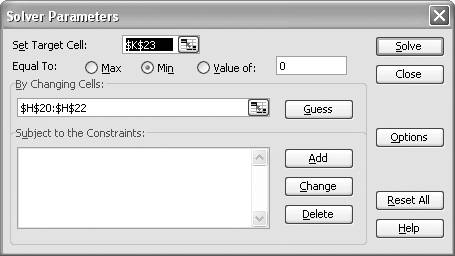Set the target cell to the cell containing the sum of squared residuals, cell K23. In this case you want to minimize the value in cell K23. Select the cells containing the x-values to vary in the By Changing Cells field. Notice that there are no constraints this time.

Upon pressing Solve, you should see the results already shown in Figure 9-16. Here again, the resulting x-values are the same as those found using the two other approaches discussed earlier.

Which approach is better depends on your preferences and the size of your problem. For larger systems I'd probably use this third methodSolver without constraintssince it would be the quickest one to set up. This is also a good example of how you might find many different, equally successful, approaches to solving the same problem in Excel.Excel Scientific and Engineering Cookbook (Cookbooks (OReilly))
ISBN: 0596008791
EAN: 2147483647
Year: N/A
Pages: 206
Authors: David M Bourg